Reasoning Practice Test IBPS Clerk Seating Arrangement Coded Inequalities set 2

(1-5) Directions: Study the following information carefully and answer the given questions...
D, E, F, H and I are seated in a circle facing the center. A, Band C are also seated in the same circle but two of them are not facing the center. F is second to the left of C. E is third to the right of A. B is third to the left of D, who is immediate neighbor of H and I. C is second to the right of D and third to the right of B.

1. Which of the following pairs is not facing the center?
a) B,C
b) A,C
c) D,E
d) B,D
e) None of these.

2. What is the position of D with respect to F?
a) 2nd to the right.
b) 3rd to the left.
c) 5th to the right.
d) Can't be determined.
e) None of these.

3. Who among the following is second to the left of B?
a) A
b) H
c) I
d) Either H or I
e) None of these.

4. If H is on the immediate right of E then what is H's position with respect to C?
a) Third to the left.
b) Third to the right.
c) Immediate right.
d) Can't be determined.
e) None of these.

5. Which of the following is/are the possible position(s) of I with respect to A? C
a) 4th to the right.
b) 2nd to the left.
c) 4th to the left.
d) Only (a) and (b)
e) All of the above.

(6-10)Directions: In the following questions, the symbols \$,@,%, © and # are used with the following meanings as illustrated below:
'P©Q' means 'P' is greater than 'Q'.
'P%Q' means 'P' is smaller than 'Q'.
'P@Q' means 'P' is either greater than or equal 'Q'.
'P\$Q' means 'P' is either smaller than or equal to 'Q'.
'P#Q' means 'P' is equal to 'Q'.
A) If only conclusion I is true.
B) If only conclusion II is true.
C) If either conclusion I or II is true.
D) If neither conclusion I nor II is true.
E) If both conclusions I and II are true

6) Statements: K@B,  B#J,  J©T
Conclusions: I. K#T  II. B@T

7) Statements: F\$M,  M@L,  L#W
Conclusions: I. W\$M  II. F@L

8) Statements: R #Q,  Q@F,  F%A
Conclusions: I. R ©A  II. R#F

9) Statements: V\$X,  X © Y,  Y% H
Conclusions: I. Y@V  II. H#V

10) Statements: M@B,  B # A,  A@F
Conclusions: I. M #A  II. B#F

1) A
2) E
3) D
4) B
5) E
6) D
7) A
8) D
9) D
10) D

Explanation:
(1-5)

(6-10)
6) K ≥ B = J >T, K > T. Hence, Conclusion I is not true. B >T. So, conclusion II is not true.

7) F ≤M ≥ L = W, M ≥ W. By conversion W ≤ M. Hence, conclusion I is true. We can't compare F and L. Hence, conclusion II is not true.

8) R = Q ≥ F < A We can't compare R and A. Hence, conclusion I is not true. R ≥ F. Hence, conclusion II is not true.

9) V ≤ X > Y < H We can't compare V and Y. Hence, conclusion I is not true. Again, H and V can't be compared. So, conclusion II is not true.

10) M ≥ B = A ≥F As M ≥ A, conclusion I is not true. Again, as B≥F, conclusion II is not true.

1.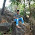plz upload solved photo

1.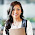Hi Sandeep,

2.Smartyzz IdeazzJuly 25, 2015 5:25 pm

Hi

For ques no 6, the ans should be (b)

Kindly check

1.Hi,
I have already provided explanation please see that
Answer is D only

3.hi mam kindly provide step wise explanation

4.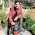Hello mam in que 8th R=F and in que R>=F is given so ans should 2 statement is true but not yy???

1.Hi Anil,
how can you say R = F and R ≥ F are same.
Can you explain it first...

5.Que 8 ans should be b.

6.please upload more questions of the similar type with solution..to gain perfection lots of practice is needed..

1.there are a lot of questions available in this blog you can try them and gain perfection all the best and keep practising

7.it says "E is third to the right of A" but ur arrangement is not satisfying it

1.My explanation diagram is showing exactly "E is 3rd to the right of A"...
what is your problem?

2.In question given that A is facing out(not center) but consider it facing center which make solution incorrect.

3.Sorry i made mistake to understand question. Your solution is correct.

8.Can you provide step by step explanation for seating arrangement question?

9.I need some explanation

10.11.The problem says that E is third to the right of A and A is facing outwards. Therefore, in the solved diagram A and E must exchange their position.

12.The problem says that E is third to the right of A and A is facing outwards. Therefore, in the solved diagram A and E must interchange their respective positions, if I am not wrong.

1.Hi Dipanjan,
who said A is facing outward?
"A, B and C are also seated in the same circle but two of them are not facing the center"
so B and C are facing outside and A is facing inside

13.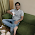Mam ... please check que. No 6 .
I think there is a problem b/w the condition 1 or say conclusion 1 & what you are considered while answering ......

1.Hi Jignesh,
Given question and conclusions are correct...
Where did you find mistake in it?

14.15.This is q. No. 6
Statements: K@B, B#J, J©T
Conclusions: I. K#T II. B@T

This is ans. For above q.6) K ≥ B = J >T, K > T. Hence, Conclusion I is not true.
K#t .... so I think we need to check for k=t....
By d way ur blog and questions r awsm .....
It definately help me to build my logical part for comp. Examination .... ....

1.Hi again,
K = T is already found false right, then why do we have to check K = T again?

16.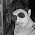Hlw .
I am very thankfull for u..I was facing prob in thi topic ....this concept really helps us ..

17.Thanks a lot...it's very usefull concept..

18.Hlw .
I am very thankfull for u..I was facing prob in thi topic ....this concept really helps us ..